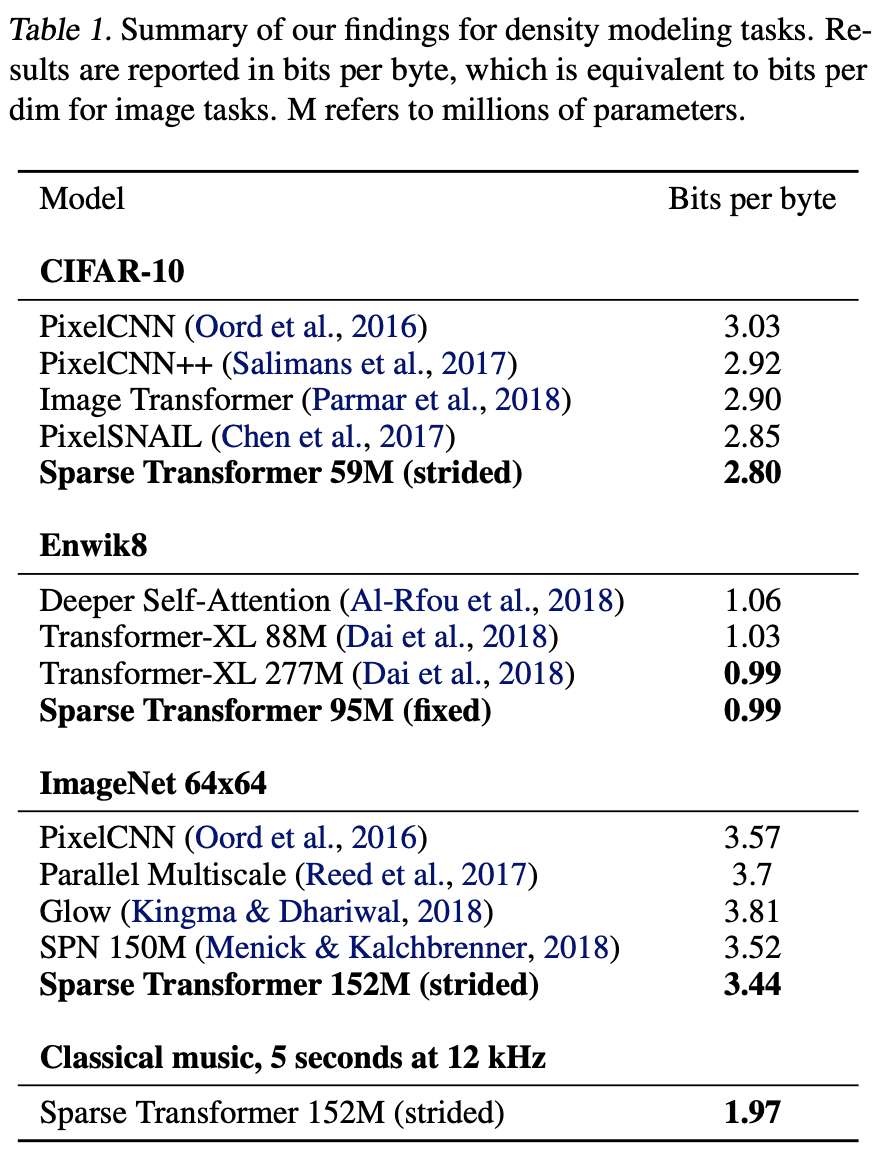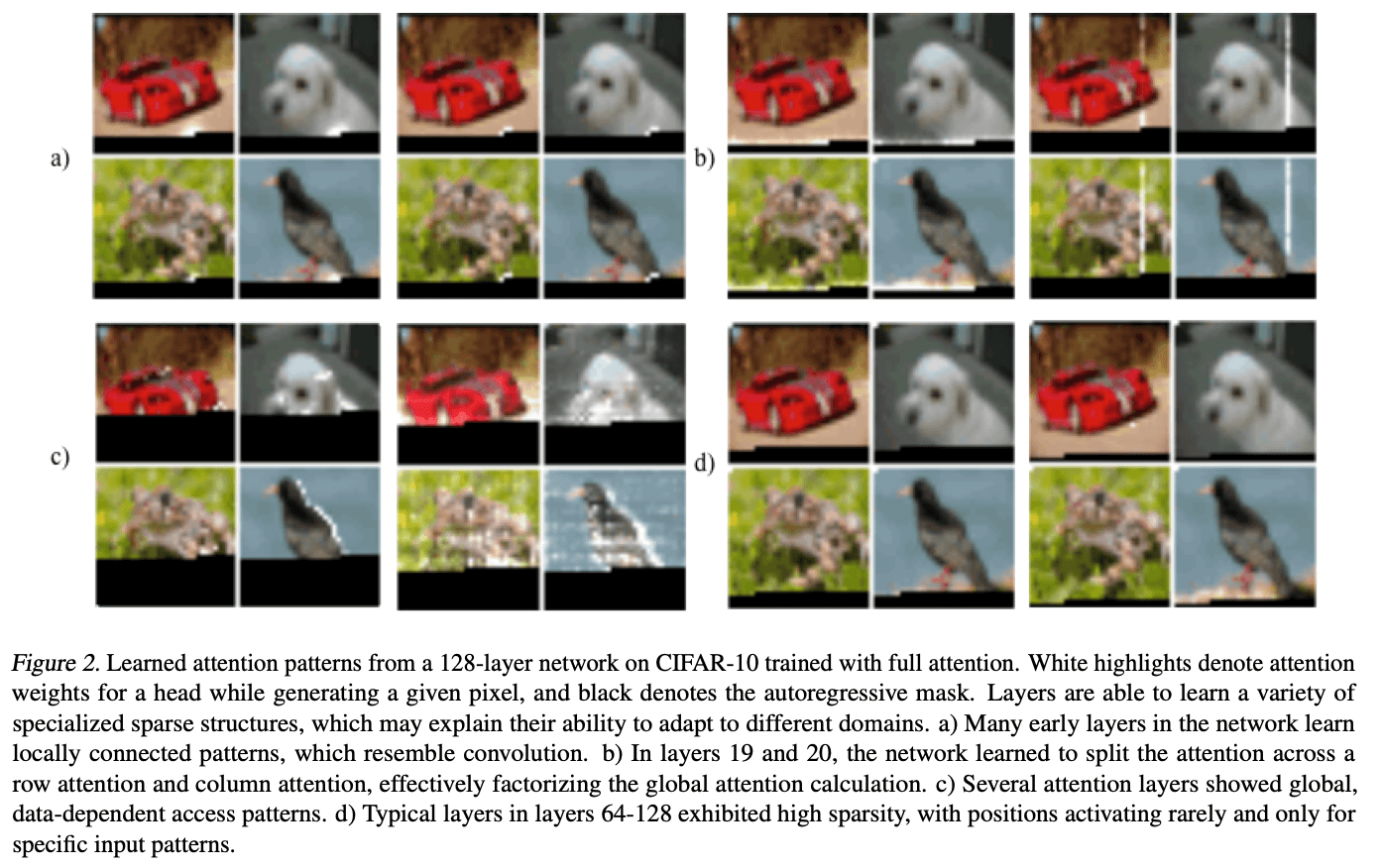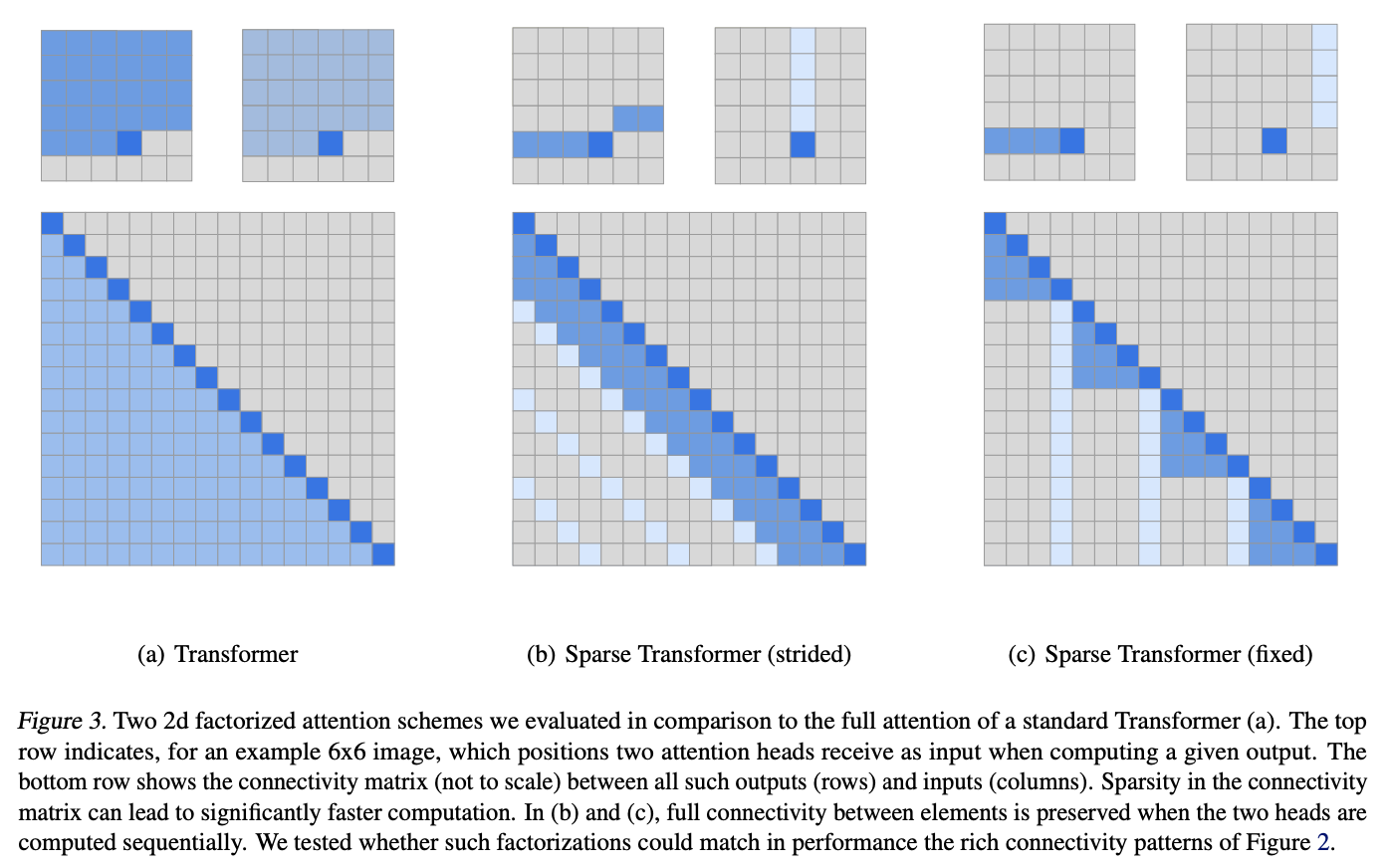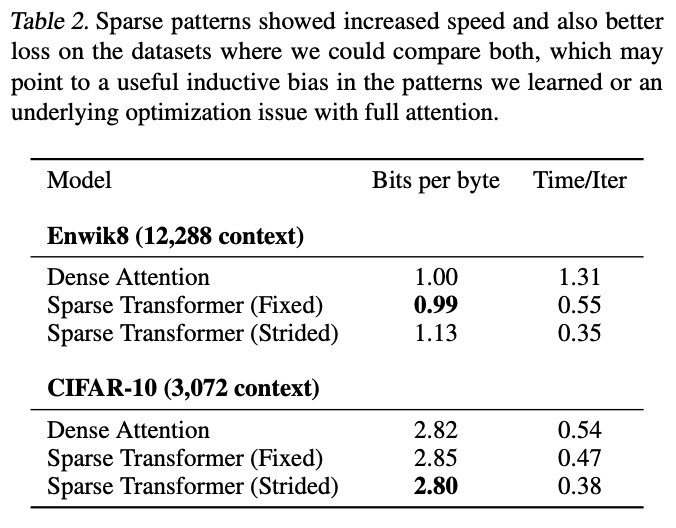# Catch up on the latest AI articles# Sparse Transformers: An Innovative Approach To The Problem Of Increasing Computational Complexity With Input Sequence Length

3 main points
✔️ Achieved computational savings by reproducing layer-by-layer features of Attention
✔️ Using three Attentions: Sliding Window Attenion, Dilated Sliding Window Attention, and Global AttentionTransformern's computational complexity was reduced by using three Attentions: Sliding Window Attention, Dilated Sliding Window Attent ion, and Global Attention.
✔️ Not only did it reduce the computational complexity, it also achieved SOTA at the time.

written by Rewon ChildScott GrayAlec RadfordIlya Sutskever
(Submitted on 23 Apr 2019)

Subjects: Machine Learning (cs.LG); Machine Learning (stat.ML)code：The images used in this article are from the paper, the introductory slides, or were created based on them.

## Summary

Sparse Transformers is an attempt to deal with the problem that the computational complexity of Transformer's self-attention is $O(n^2)$ and that memory usage increases rapidly when long sentences are inserted.

## What is the problem with Transformer?

The computational complexity of a transformer increases quadratically with the input sequence. This results in very long computation times and memory usage.

This is because of Scaled Dot-Product Self-Attention, a key component of Transformer. To begin with, Scaled Dot-Product Self-Attention calculates Attention using a query and key-value pairs. In other words, the formula for calculating Scaled Dot-Product Self-Attention uses the query, key, and value ($Q,K,V$) to compute

$$Attention(Q, K, V) = softmax(\frac{QK^T}{\sqrt{d_k}}V)$$

The product of the query and the value (Q, V) is the square of the document length ($n$). The product of the query and the value (Q, V) is the square of the document length ($n$). Therefore, given 2046 tokens as input, the matrix size used in the Attention calculation is 2024*2024, which means that a matrix with approximately 4.1 million elements must be processed in the Attention calculation. This is required for the batch size, which is computationally expensive, and puts pressure on memory capacity.

Therefore, this paper, Sparse Transformers, addresses this Transformer problem of increasing computational complexity as the input sequence is squared.

## Results using Sparse Transformers

First, let us see how much memory usage has been reduced as a result of using Sparse Transformers. Specifically, as shown in Table 1 below, Sota was achieved in all domains (image, language, and audio). We also describe the actual environment used for the tests.### CIFAR-10

To begin with, CIFAR-10 itself is a 32 x 32 pixel color image, so the input sequence length for a single image is 32 x 32 x 3 = 3072 bytes. This is learned with Strided Sparse Transformers. Parameters such as two heads, 128 layers, and $d$=256 are trained in a half-size feedforward network. The learning rate is 0.00035, the dropout rate is 0.25 until the validation error stops decreasing, and the number of epochs is 120 epochs.

We used 48,000 samples of data for training and 2,000 samples for validation to evaluate the performance of the model. The result is 2.80, which is better than the 2.85 achieved by Chen et al.

### Enwik8

Evaluating Sparse Transformers on the Enwik8 dataset for longer sequence input, the Enwik8 dataset has a sentence length of 12,228, which is a long sequence input.

The training is done using 30 layers of Fixed Sparse Transformers with the first 90 million tokens, and the last 10 million tokens are for validation and testing. The training parameters are 8 heads, $d$=512, 128 strides with a dropout rate of 0.40, c=32 and 80 epochs.

As a result, we achieved 0.99, exceeding the la 1.03 of Transformer-XL (Dai et al., 2018), which was the Sota at the time of publication and of similar size.

### ImageNet64×64

ImageNet and CIFAR-10 are from the same set of image systems, but the difference is the length of the input sequences; ImageNet 64x64 must handle sequences that are four times longer than those in CIFAR-10. Therefore, it is a test to see if strided sparse transformers can retain long-term memory well.

Experiments using ImageNet 64×64 were trained with 16 Attention Heads and 48 layers of strided Sparse Transformers with $d$=512 for a total of 152 million parameters. The parameters appear to have been validated with a stride of 128, a dropout rate of 0.01, and an epoch count of 70 epochs.

The resulting reduction is 3.44 bits per dim compared to the previous 3.52 (Menick & Kalch- brenner, 2018). Visual evaluation also shows that the generation captures the long-term structure in most images.

### Classical music

They trained the model on a classical music dataset published by (Dieleman et al., 2018) to test how well the Sparse Transformer handles very long contexts (more than 5 times longer than Enwik8). However, the authors also point out that the details of the dataset are not available, so they cannot compare their results with existing studies.

However, using the classical music dataset published by (Dieleman et al., 2018), they claim to have been able to perform self-attention at many time steps, despite having very few parameters. Indeed, you can listen to it at:
https://openai.com/blog/ sparse-transformer

## What did you do with Sparse Transformers?

The following is an introduction to Sparse Transformers, starting with how the idea of Sparse Transformers came about and how it was actually used to reduce the computational complexity.

### Understanding of existing Attention

When considering Sparse Transformers, it is important to understand where existing Attention is directed. The authors have also visualized where existing Attention is directed, layer by layer. Specifically, Figure 2 below shows the results of training CIFAR-10 on a network with 128 layers.As a result, the

（In the initial layer of the network shown in (a), we can see that attention is directed to the immediately preceding information highlighted in white.

（In layers 19 and 20 shown by (b), Attention is directed in the row and column directions. This can be considered as effective learning of bandwidth-like features.

（What (c) shows is that Attention is scattered throughout the image.

(d) The 64th to 128th layers show sparsity, and it is difficult to tell where the Attention is directed.

Sparse Transformers attempts to design more efficient Attention based on these existing Attention features.

### Sparse Transformers Attention Devices

Attention in Sparse Transformers uses the Attention features in (b) and (c) above.

Following the illustrations in the paper, we consider the example of a 6x6 image.・(a) Transformer (Full Attention)

Attention in Figure 3(a) is the attention used by the normal transformer, which directs the attention to all positions before itself. The computational complexity is $O(n^2)$.

・(b) Sparse Transformers (Strided Attention)

Attention in Figure 3(b) divides the head into two parts. The blue one does not pay attention to all the elements before its own position, but only to the last three. The light blue one focuses Attention on every third element.

In short, the blue color directs Attention horizontally, while the light blue color directs Attention vertically. This reproduces the Attention pattern in state (b) of the previous image.

Experiments have also shown that such Attention is effective for data with periodic trends, such as images and audio.

・(c) Sparse Transformers (Fixed Attention)

In Figure 3(c), Strided Attention in (b) uses relative position to direct Attention, while Fixed Attention in (c) adds an absolute position element to determine the position to direct Attention. In contrast, Fixed Attention in (c) adds an absolute position element to determine the position to direct Attention. Thus, the element in blue directs Attention to some elements in front of itself, while the element in light blue directs Attention to all elements vertically at regular intervals.

Experiments have shown that such Attention is effective for textual information such as sentences.

### Strided Attention and Fixed Attention in Sparse Transformers

Results of experiments using Strided Attention and Fixed Attention on image and text data.

- Strided Attention is valid for image datasets and
- Fixed Attention is valid for text datasets

The results of this study show that## Summary

In Sparse Transformers, we investigated the behavior of the existing Attention layer by layer, and were able to reproduce the behavior of row and column orientation, as well as the behavior of looking evenly across the entire image, which we considered important, with two newly introduced architectures, Strided Attention and Fixed Attention. The two newly introduced architectures, Strided Attention and Fixed Attention, were able to reproduce these behaviors, solving the old transformer's computation problem and achieving several of the Sota levels at the time of publication.

There have been other attempts to optimize the computational complexity of transformers since Sparse Transformers. Please refer to other Longnet and other sources if you wish.

If you have any suggestions for improvement of the content of the article,